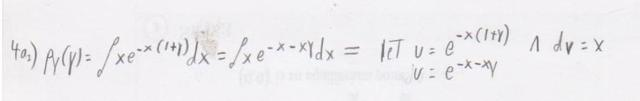# Difficulty With Integration by Parts

Shoney45

## Homework Statement## The Attempt at a Solution

What I am unsure of is how to find the derivative of u. Since the original integral is integrating with respect to y, should I be finding the derivative of u with respect to y, and treat the x's as contants?

Roni1985
I think it's possible to solve it in terms of x

let u=x and dv=e^(-x(1+y))

then,
du=1
and v= [e^(-x(1+y))]/(-(1+y))

I think it should work

if you want let
u=e^(-x(1+y))

du= -(1+y)*e^(-x(1+y))

you treat y as a constant and x as a variable
(I am assuming you want to integrate in terms of x)

Shoney45
Alrighty then - I'll get to work. Thanks for the direction.

jav
I think it's possible to solve it in terms of x

let u=x and dv=e^(-x(1+y))

then,
du=1
and v= [e^(-x(1+y))]/(-(1+y))

Actually du = dx, also dv=e^(-x(1+y))dx

You can simplify this problem, by performing a w substitution at the beginning ie. consider w = -x*(1+y). It will make the integration by parts less of a headache.